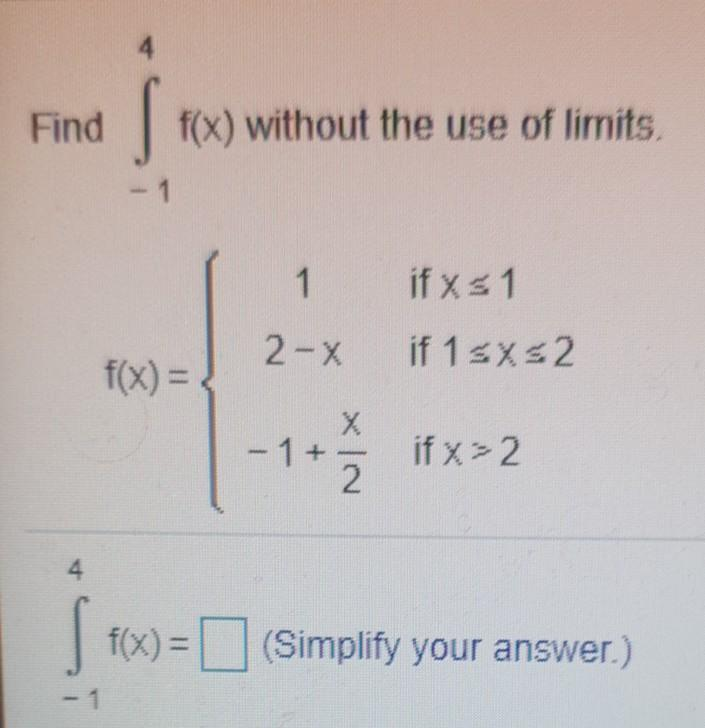### Create an Account

Already have account?

### Forgot Your Password ?

Home / Questions / Find f (x) without the use of limits. - 1 1 if x1 2-X if 1sx52 f(x) = X - 1 + + if x=2 2. ...

# Find f (x) without the use of limits. - 1 1 if x1 2-X if 1sx52 f(x) = X - 1 + + if x=2 2. 4 tex) = (Simplify your answer.) )

Find f (x) without the use of limits. - 1 1 if x1 2-X if 1sx52 f(x) = X - 1 + + if x=2 2. 4 tex) = (Simplify your answer.) )Apr 15 2021 View more View Less

#### Answer (Solved)Subscribe To Get Solution